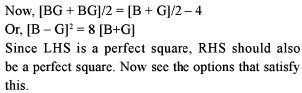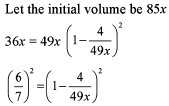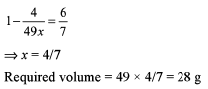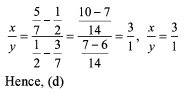Courses

# Arun Sharma Test: Averages- 3

## 15 Questions MCQ Test Quantitative Aptitude (Quant) | Arun Sharma Test: Averages- 3

Description
This mock test of Arun Sharma Test: Averages- 3 for Quant helps you for every Quant entrance exam. This contains 15 Multiple Choice Questions for Quant Arun Sharma Test: Averages- 3 (mcq) to study with solutions a complete question bank. The solved questions answers in this Arun Sharma Test: Averages- 3 quiz give you a good mix of easy questions and tough questions. Quant students definitely take this Arun Sharma Test: Averages- 3 exercise for a better result in the exam. You can find other Arun Sharma Test: Averages- 3 extra questions, long questions & short questions for Quant on EduRev as well by searching above.
QUESTION: 1

### The average marks of the girls in a class is equal to the number of boys and the average marks of boys is equal to the number of girls. If the class average is 4 less than the average of both the boys’ and the girls’ average marks, what will be the number of students in the class?

Solution:QUESTION: 2

### A, B, C, D and E are five consecutive integers and the average of these five numbers is less than 1/4th of A.Then A is

Solution:

1stly, it is given that a, b, c, d and e are 5 consecutive integers. So,
b=a+1, c= a+2, d=a+3, e=a+4
2ndly, it is given that
(a+b+c+d+e)/5<a/4
=>{a+a+1+a+2+a+3+a+4)/5<a/4
=>4(5a+10)<5a
=>20a+40<5a
=>20a-5a<-40
=>15a<-40
=>a<-40/15
As a is less than a negative number, so 'a' is also a negative number.

QUESTION: 3

### I was bom 30 years after my father was born. My sister was bom 25 years after my mother was born. The average age of my family is 26.25 years right now. My sister will get married 4 years from now and will leave the family. Then the average age of the family  will be - 107/3 years. What is the age o f my father?

Solution:

Let present age of father = x year and present age o f mother = y year therefore present age of son = (x -30) and present age o f daughter = (y-25)

Sum of their ages = 4 x 26.25 = 105 years i.e ., x + >y + x - 3 0 + >y - 2 5 = 105

2x + 2y = 160

x + T = 80   ...(1)

After 4 years, their total ages will be (excluding the daughter)

x+4+y+4+ x-30+4 = 107

2x + y - 18 = 107

2x + y = 125         ...(2)

Solving (1) and (2)

x = 45

QUESTION: 4

India is supposed to send its boxing team for Olympics in each of the following 10 weight group divisions.

A (48 kg -52 kg)        B (52 kg - 56 kg)

C (56 k g - 6 0 kg)     D (60 k g - 6 4 kg)

E (64 kg -68 kg)        F (68 kg - 72 kg)

G (72 k g - 76 kg)    H (76 k g - 80 kg)

I (80 kg - 84 kg)       J (84 kg - 88 kg)

After selecting one player from each group, their average weight comes out to be 68 kg. if one of the players named X leaves

If X leaves the team, and two new players join the group, then their average weight increases to 68 kg. These players can be from group....

Solution:

Total weight of 11 players ( 68 x 11) kg = 748 kg

Increase in weight = (748 - 598.5) kg = 149.5

QUESTION: 5

In continuation with previous question, the average of all the groups together, which group contributes most in overall average?

Solution:

Exact weight of players are not known hence (d)

QUESTION: 6

The average age of Mr and Mrs Sinha at the time of their marriage in 1972 was 23 years. On the occasion of their anniversary in 1976, they observed that the average age of their family had come down by 4 years compared to their average age at the time of their marriage. This was due to the fact that their son Vicky was born during that period. What was the age of Vicky in 1980?

Solution:

Sum of ages of Mr and Mrs Sinha in 1972 = 46 years Sum of age of their family in 1976 = 19 x 3 = 57 years

Sum of ages of Mr & Mrs Sinha in 1976 = (46 + 8) years = 54 years

their son’s age in 1976 = (57 - 54) years = 3 years

QUESTION: 7

Sachin Tendulkar has a certain batting average N (a whole number) in his career of 86 innings. In the 87th inning, he gets out after scoring 270 runs which increases his batting average by a whole number. The batting average is calculated by dividing the total number of runs scored by the total number of innings played by the player. How many values of his new average is/are possible?

Solution:

Total number of runs scored till 86th inning = 86 N Now, 86 N + 270 = 87 (N + S), where S is the increase in batting average.
Different values of S possible now S = 0, 1, 2 and 3.

QUESTION: 8

Read the passage below and solve the questions based on it.
The average score of a batsman for a certain number of innings was 21 75 per inning. He played 3 innings more and scored 28. 34 and 37 runs respectively, thus increasing his average by 1.25.

Q.

What was his total score?

Solution:

Total score = 21.75 x 24 + 28 + 34 + 37 = 621

QUESTION: 9

Rakesh has a stock of 1000 litres of pure milk. He decides to decrease the concentration to half, using the method of successive dilution, replacing 200 litres of contents by water, in each step of dilution. After the fourth dilution, he realizes that the concentration is less than the required value. Each of the contents removed during the dilution process is stored separately. He also has ample supply of water. Will he be able to make the mixture of desired concentration?

Solution:

It is possible because each contents removed during the dilution process is stored separately.

QUESTION: 10

Four gallons are drawn from a case full of wine. It is then filled with water. Four gallons of mixture are again drawn and the cask is re-filled with water. The ratio of the quantity of wine now left in the cask to that of the mixture in it is 36:49. How much does the cask hold?

Solution:QUESTION: 11

There are three vessels A, B and C having a mixture of milk and water. Concentration of milk in the vessels is C1? C2 and C3 respectively. When a part of vessel A is replaced with the mixture in vessel B, then the concentration of milk in the final mixture is less than Cr However, when vessels B and C are mixed in the ratio of 1:1, then the quantity of milk in the final mixture is double than the quantity of milk in the vessel B. Which of the vessels is having the mixture of maximum concentration of milk?

Solution:

When a part of vessel A is replaced with the mixture in vessel B, then the concentration of milk in the final mixture is less than C- I t im p lie s th a t C1 > C2 When vessels B and C are mixed in the ratio 1:1, then the quantity of milk in the final mixture is double than the quantity of milk in the vessel B - It implies that C2 = C3
Hence C1 is greatest

QUESTION: 12

In two alloys, the ratios of copper to zinc is 5:2 and 3:4. How many kilograms of the first alloy and the second alloy respectively should be melted together to obtain 28 kg of a new alloy with equal copper and zinc?

Solution:

Let x and y kg of 1 st Alloy and 2nd Alloy are takenQUESTION: 13

If the average marks of 17 students in a class is A. The marks of the students when arranged in either an ascending or a descending order was found to be in arithmetic progression. The class teacher found that the students who were ranked 3rd, 7th, 9th, 11th, 15 th had copied in the exam and hence got all of them rusticated. The average of the remainder of the class was B. Then

Solution:

This question is one standard example of the definition of average in terms of surplus and deficit of the values. (Refer to the definition given) Now, what we were expected to mark here was the fact that whatever is the average (in this case, it is A), surplus generated by the marks of 3rd student will be same as deficit incurred due to 15th student. So, rusticating both of them is not going to create any difference on average marks of the class (remember marks are in AP). And similar will be the impact of rusticating 7th student and 11th student and then finally 9th student.
So, A = B

QUESTION: 14

Illara, Puttu and Munchun had three bottles containing equal amounts of orange juice, mango juice and water respectively. Since Illara and Puttu wanted to dilute their drinks, Munchun gave one-fourth of the water that she had, to each of them. After mixing their bottles well, each of them gave to Munchun the same quantity of the solution that they had borrowed from her. Which of the following statements is true? i. The concentration of water in Munchun’s drink is more than the concentration of orange juice in Illara’s drink.ii. The concentration of orange juice in Illara’s drink is more than the concentration of mango juice in Puttu’s drink. iii. The concentration of orange is more than the concentration of mango in Munchun’s drink.

Solution:

Basically in these types of situations, we should start with an attempt of refuting the statements given. The statement that cannot be refuted will be true, otherwise false.
Checking statement i, since Munchun is donating V2 of water from her bottle and Illara is donating only V* of mixture from her bottle, concentration of water in Munchun’s drink will be less than the concentration of orange juice in Illara’s drink. So, statement i. is not true.
Checking statement ii, since Illara and Puttu are receiving and then donating the same amount of liquid, so concentration of orange juice in Illara’s drink will be equal to the concentration of mango juice in Puttu’s drink. So, statement ii. is also not true.
Checking statement iii, concentration of orange will be equal to the concentration of mango in Munchun’s drink. So, statement iii is also wrong.
Hence option (d). None of these is the answer.

QUESTION: 15

We write down all the digits from 1-9 side by side. Now. we put "+" between as many digits as we wish to. so that the sum of numbers become 666.It is explained below: 1  2  3  4  5  6  7  8  9 = 666`

Now suppose we put plus signs al following places: 12 + 3 5 + 67 + 89 = 513` Since there are four numbers, so the average can be calculated by dividing the bum by 4. What is the average if the sum is 666?

In the above question, what is the average if the sum is 261?

Solution:

261 is possible only if we take 123 +45 + 6 + 78 + 9 So, the average will be 261/5 = 52.2